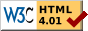## Dokumentation zu: [](E)``` SYNOPSIS mixed arr[index] int str[index] mixed * arr[from .. to] string str[from .. to] DESCRIPTION Return one element from a string/array (first form), or a slice (substring resp. subarray) of the string/array (second form). The indexes , and are numbered 0 to strlen(str)-1 resp. sizeof(arr)-1 . If an index is written 'value', the value is counted from the end of the string/array if it is negative (starting with -1 for the last element), and from the beginning if it is positive (starting with 0 for the first element). If is omitted, it defaults to the beginning of the string/array. If is omitted, it defaults to the beginning of the string/array. In the first form, the must be within the bounds of the string/array, or a runtime error occurs. In the second form, the indexes will be fitted to the bounds of the string/array. If is greater than , or both outside the bounds, an empty string/array ("" resp. ({})) will be returned. The closure notation is straightforward: [index] -> ({'#[, arr, index }) [ ({'#[<, arr, index }) [>index] -> ({'#[>, arr, index }) [from..to] -> ({'#[..], arr, from, to }) [ ({'#[<..], arr, from, to }) [from.. ({'#[..<], arr, from, to }) [ ({'#[<..<], arr, from, to }) [>from..to] -> ({'#[>..], arr, from, to }) [from..>to] -> ({'#[..>], arr, from, to }) [>from.. ({'#[>..<], arr, from, to }) [to] -> ({'#[<..>], arr, from, to }) [>from..>to] -> ({'#[>..>], arr, from, to }) EXAMPLES foo = ({ 1, 2, 3, 4 }); str = "test"; foo -> 1 str -> 'e' == 101 foo[1..2] -> ({ 2, 3 }) str[1..2] -> "es" foo[2..1] -> ({ }) str[2..1] -> "" foo[0..<2] -> ({ 1, 2 }) str[0..<2] -> "tes" foo[..<2] -> ({ 1, 2 }) str[..<2] -> "tes" foo[<3..] -> ({ 2, 3, 4 }) str[<3..] -> "est" foo = 5 -> foo == ({ 1, 5, 3, 4 }) foo[1..2] = ({ 5, 6, 7 }) -> foo == ({ 1, 5, 6, 7, 4 }) foo[1..2] = ({ }) -> foo == ({ 1, 4 }) str = 'a' -> str == "tast" str[1..2] = "bar" -> str == "tbart" str[1..2] = "" -> str == "tt" HISTORY slice_array() is the old form of the [] operator on arrays. extract() is the old form of the [] operator on strings. Both ARE NO LONGER SUPPORTED and should not be used anymore! The syntax for ``counting from last element'' has changed between versions 3.1.J and 3.1.K from ``-1'' to ``<1''. foo[0..-1] is now an empty string resp. array. LDMud 3.3 introduced the '>' indexing method. SEE ALSO member(E), sizeof(E) ``` Die Seite ist auch in Deutsch vorhanden.

Start » Magierhandbuch » Docu » Efun » [] Letzte Generierung: 25.04.2021, 01:58mud@wl.mud.de